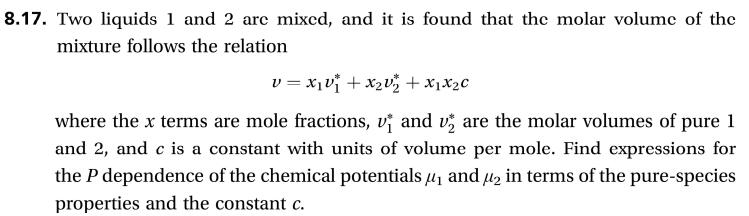# 8.17. Two liquids 1 and 2 arc mixcd, and it is found that the molar volumc of themixture follows the relationvxix2vz x1x2cand v are the molar volumes of pure 1where the x terms are mole fractions,and 2, and c is a constant with units of volume per mole. Find expressions forthe P dependence of the chemical potentials and in terms of the pure-speciesproperties and the constant c.

Question
3 views

This problem is (8.17) from a book  "Thermodynamics and Statistical Mechanics An Integrated Approach by M. Scott Shell"help_outlineImage Transcriptionclose8.17. Two liquids 1 and 2 arc mixcd, and it is found that the molar volumc of the mixture follows the relation vxix2vz x1x2c and v are the molar volumes of pure 1 where the x terms are mole fractions, and 2, and c is a constant with units of volume per mole. Find expressions for the P dependence of the chemical potentials and in terms of the pure-species properties and the constant c. fullscreen
check_circle

Step 1

Where x denotes mole fraction of liquids (1 and 2) in the solution

ʋ1* denotes molar volume of pure 1

ʋ2*denotes molar volume of pure 2

C is constant (volume/mol)

Step 2

From the fundamental thermodynamic relation in terms of Gibbs free energy and use Maxwell relation to find out pressure dependence of chemical potential

For mixture thermodynamic relation is written as follows:

Step 3

From Maxwell relation equati...

### Want to see the full answer?

See Solution

#### Want to see this answer and more?

Solutions are written by subject experts who are available 24/7. Questions are typically answered within 1 hour.*

See Solution
*Response times may vary by subject and question.
Tagged in

### Chemical Engineering